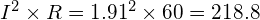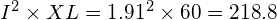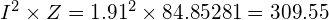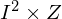# How to improve Power Factor? The Basic Principle

How to improve Power Factor? The Basic Principle 1024 576 Gaurav J

In this tutorial, I’ll be talking about, the very basic principle for improving power factor. Which will help you to understand how you can improve power factor. So, let’s start.

If you are looking for a tutorial about different ways of improving power factor then, this tutorial is not for you. In this tutorial, we’ll be looking at the very basic principle of how you can improve the power factor. And the ways to do it, that we will see in our next tutorial of power factor series.

Recommend Readings before moving on in this tutorial:

## The Basic Principle

Let’s say, I have a zero. And I want to keep that zero constant. But, someone added +7 in it, and now my number is changed to +7.

#### 0 + 7 = +7

So, to get my original number again what I have to do? I hope you have guessed it right, yes, I have to add equal & opposite number with it. So, I added -7, and I got my original number back i.e. zero!

#### (+7) +( -7) = 0

Well, if you understood this simple math, you have understood how you can improve power factor, now I only need to explain the concept.

Now, if your system’s total impedance is equal to the total resistance then it means that, your power factor is good, & no reactive power is consumed. But, as soon as you add load to it, the load, most of the time is inductive load. Therefore, your total impedance is no longer equal to your total resistance. Which means that you are consuming reactive power & the power factor is poor.

Now to get back to your original position back i.e. Total resistance = Total impedance, you have to add equal & opposite amount of reactive power to the system. Yes, it is as simple as that.

So, what kills inductive reactance? Yes, capacitive reactance. So, you have to add equal & opposite amount of capacitive reactance in the circuit. The effect of this two equal & opposing reactances i.e. inductive & capacitive will bring the circuit’s Total impedance = Total resistance.

## Understand more clearly

Consider the example shown to understand more clearly.

Consider that we have 230V AC supply connected to a load as shown. Current flowing through the circuit is 1.91 amps.

• Active Power =watts
• Reactive Power =VAR
• Apparent Power =VA

The active power in this circuit is 218.8 watts, reactive power is also 218.8 VAR, and the apparent power is 309 VA. So, if we calculate power factor of this circuit we get power factor = 0.7 (PF = Active power/Apparent power). Now, to improve the power factor of this circuit, as we have seen, we need to inject equal & opposite amount of reactive power in it. As the reactive power is inductive, we’ll add a capacitor in parallel with the load. Now let’s see, what this capacitor will do with our circuit.

With the addition of capacitor, the total impedance of the circuit is reduced to almost 61 ohms (assume) which was 84.85 ohms in previous case (without capacitor). And hence the apparent power will now change to 222.53 VA (). If we calculate the power factor now, we’ll get PF = 0.98.

Let’s understand what just happened here. The below power triangle shows the different types of powers in the circuit, without capacitor.

Here we can see the inductive reactive power 218.8 VAR. To improve the power factor we need to add equal & opposite amount of reactive power. So, we added the capacitor.

Reactive power we are getting from capacitor is let say is X VAR. So, this positive & negative power will cancel out each other, and the resultant power triangle will look like this.

(218.8 – X) VAR reactive power now remains in the circuit, and which has caused improvement in power factor. There are different ways you can use to inject the opposite reactive power is the system. These different ways we’ll see in our next tutorial.

If your circuit is predominantly inductive, then the power factor of the circuit is lagging, as the current in case of inductive load lags behind the voltage. Similarly, if the load is capacitive then the power factor of the circuit is leading, as the current leads the voltage in case of capacitive load.

So, I hope you guys have understood the very basic of how you can actually improve power factor.###### Gaurav J

Electrical Engineer. Content Creator. Currently working with a High & Extra High Voltage Switchgear Industry.

All stories by: Gaurav J